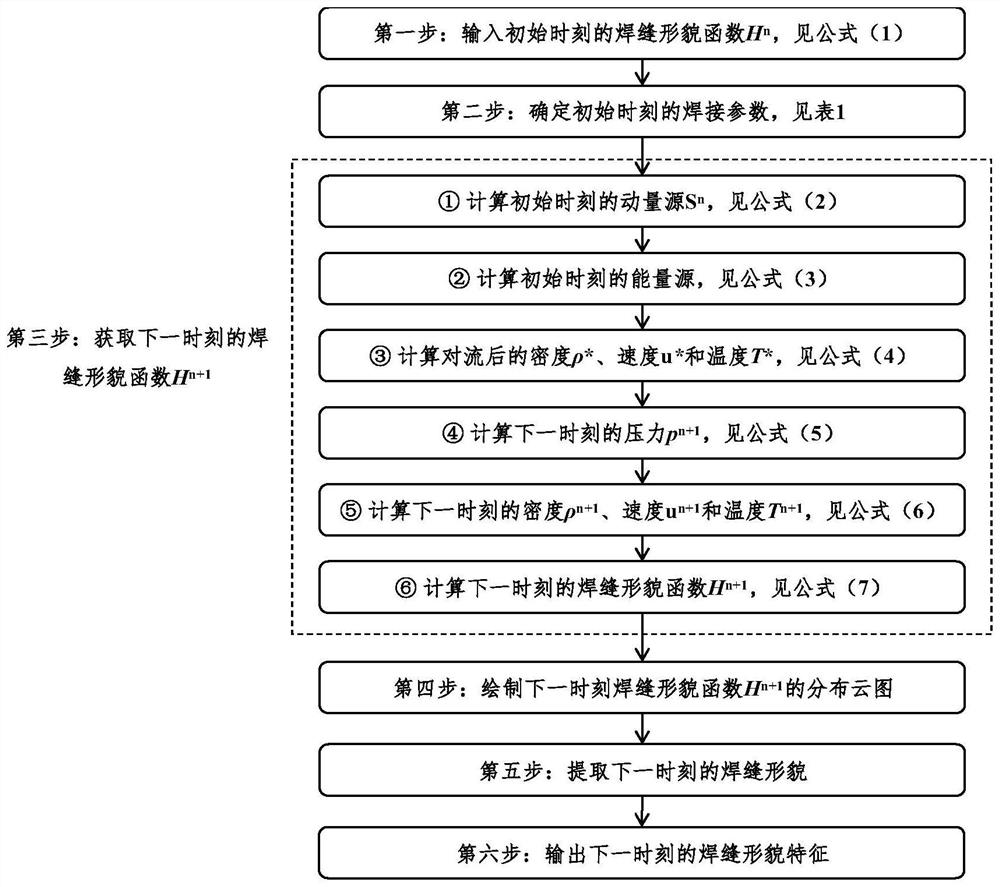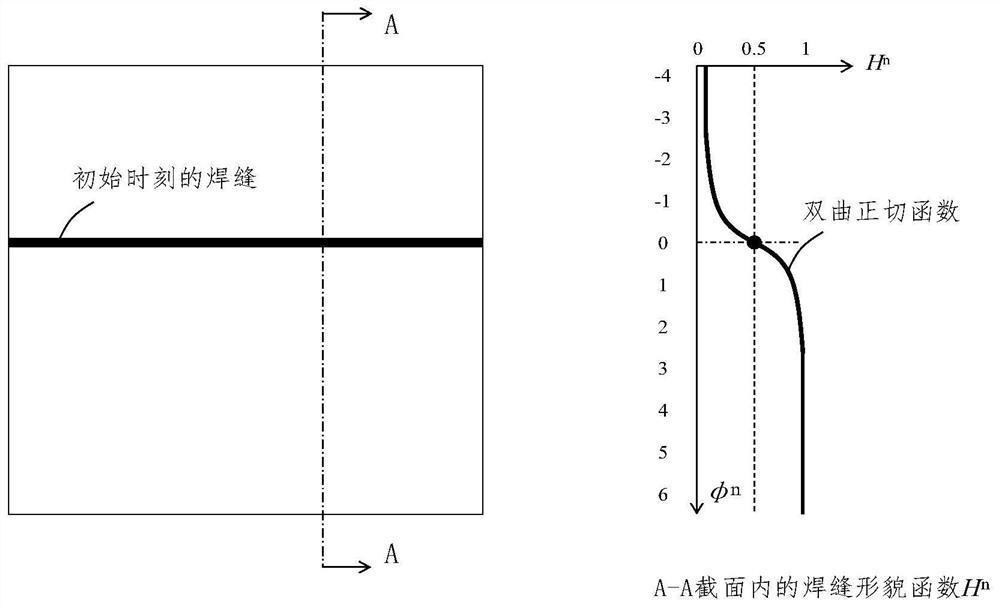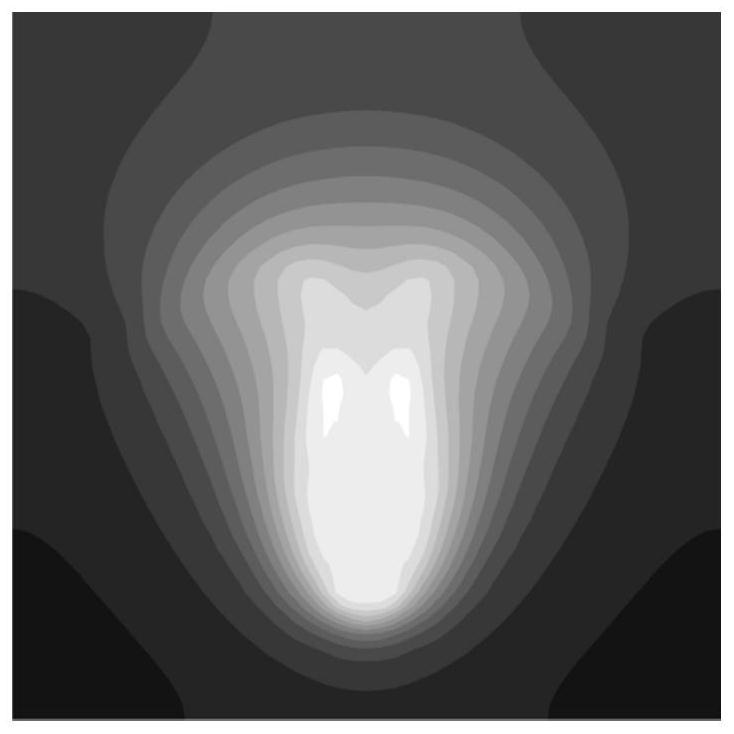# High-precision weld shape prediction method suitable for myriawatt laser welding

## A technology of laser welding and prediction method, which is applied in the direction of instruments, complex mathematical operations, design optimization/simulation, etc., can solve the problem of low prediction accuracy of weld shape, and achieve high pressure calculation accuracy, good physical conservation, and stable calculation good sex effect

Pending Publication Date: 2022-08-02
CHANGSHU INSTITUTE OF TECHNOLOGY
0 Cites 0 Cited by

## AI-Extracted Technical Summary

### Problems solved by technology

 In order to solve the problem of low weld seam shape prediction accuracy in 10,000-watt laser welding, the p...
View more

### Method used

Can't consider molten pool and plume coupling behavior and then cause the low problem of 10,000-watt laser welding seam shape prediction accuracy for existing weld shape prediction method, the present invention adopts the compressible two-...
View more

## Abstract

The invention discloses a high-precision weld joint morphology prediction method suitable for myriawatt-level laser welding, and aims to solve the problem that the weld joint morphology prediction precision of myriawatt-level laser welding is low due to the fact that a weld pool and plume coupling behavior cannot be considered in an existing weld joint morphology prediction method. According to the method, a compressible two-phase flow numerical calculation method based on pressure is adopted to solve the coupling behavior of the molten pool and plume, and therefore high-precision prediction of the myriawatt-level laser welding seam morphology is achieved. Firstly, a welding seam morphology function and welding parameters at the initial moment are input; secondly, a compressible two-phase flow numerical calculation method based on pressure is adopted to obtain a welding seam morphology function at the next moment; and drawing a welding seam morphology function at the next moment, and extracting welding seam morphology and welding seam morphology characteristics. Compared with an existing weld joint morphology prediction method, the weld pool and plume coupling behavior in myriawatt-level laser welding can be accurately calculated, the algorithm is simple and easy to implement, the calculation efficiency is high, the physical conservation is good, and high-precision prediction of the myriawatt-level laser welding weld joint morphology can be achieved.

Application Domain

Design optimisation/simulationManufacturing computing systems +1

Technology Topic

PhysicsWeld seam +6

## Image

•••## Examples

• Experimental program(1)

### Example Embodiment

 The present invention will be further described below with reference to the accompanying drawings and embodiments.
 Aiming at the problem that the existing welding seam morphology prediction method cannot consider the coupling behavior of the molten pool and the plume, which leads to the low prediction accuracy of the 10,000-watt laser welding seam morphology, the present invention adopts the pressure-based compressible two-phase flow numerical calculation method to solve the problem. The coupling behavior of molten pool and plume can realize high-precision prediction of weld seam morphology of 10,000-watt laser welding.
 combine figure 1 , a method for predicting high-precision weld seam morphology suitable for 10,000-watt laser welding according to the present invention, comprising the following steps:
 Step 1: Input the weld profile function at the initial moment
 The present invention uses the hyperbolic tangent function to represent the weld shape H n , the weld topography is defined as the 0.5 isosurface of the hyperbolic tangent function. The weld profile function H at the initial moment n See formula (1):

 where Δx min is the minimum grid size, φ n is the distance from each point in the workpiece section to the weld at the initial moment, and tanh is the hyperbolic tangent function, such as figure 2 shown.
 Step 2: Determine the welding parameters at the initial moment
 The welding parameters at the initial moment are shown in Table 1:
 Table 1 Welding parameters at the initial moment


 Step 3: Obtain the weld profile function H at the next moment n+1
 The present invention proposes a pressure-based numerical calculation method for compressible two-phase flow to solve the weld topography function H at the next moment. n+1 , the specific implementation process is as follows:
 ①Calculate the momentum source S at the initial moment n , see formula (2).

 in, represent the momentum sources caused by inertia, surface force, and body force, respectively, is the gradient operator, · is the divergence operator, superscript T stands for matrix transpose, ρ n , u n , T n , μ, α, T env , σ, A, B 0 , m, L, T s Given by Table 1, g is the gravitational acceleration constant, k b is the Boltzmann constant.
 ②Calculate the energy source Q at the initial moment n , see equation (3).

 in, is the gradient operator, · is the divergence operator, ρ n , u n , T n , C p , λ, I, r 0 Given by Table 1, Given by formula (2), r is the distance from each point to the center of the laser spot.
 ③ Calculate the density ρ after convection * , speed u * and temperature T * , see equation (4).

 in, is the gradient operator, is the divergence operator, Δt is the calculation time step, u n , T n , ρ n , u n , T n given in Table 1.
 ④ Calculate the pressure p at the next moment n+1 , see equation (5).

 in, is the gradient operator, · is the divergence operator, Δt is the calculation time step, ρ*, u*, T* are obtained from formula (4), C V Given by Table 1, R is the ideal gas constant.
 ⑤ Calculate the density ρ at the next moment n+1 , speed u n+1 , temperature T n+1 , see equation (6).

 in, is the gradient operator, · is the divergence operator, Δt is the calculation time step, ρ*, u*, T* are given by formula (4), C V Given by Table 1, R is the ideal gas constant.
 ⑥ Calculate the weld profile function H at the next moment n+1 , see equation (7).

 in, is the gradient operator, Δt is the calculation time step, H n Given by formula (1), u n+1 is given by equation (6).
 Among them, the gradient operator in formula (2) to formula (7) and the divergence operator ·The FiniteVolume Method is used for discretization to ensure strict physical field conservation.
 Formula (5) can be solved by methods such as the Success Over Relaxation Method, the Conjugate Gradient Method or the Fast Fourier Transform.
 Step 4: Draw the weld profile function H at the next moment n+1 distribution cloud map.
 According to the weld topography function H at the next moment n+1 , draw the distribution cloud map of the weld topography function, such as image 3 shown.
 Step 5: Extract the weld profile at the next moment.
 According to the weld topography function H at the next moment n+1 , extract H n+1 The 0.5 isosurface is used as the weld morphology at the next moment, such as Figure 4 shown.
 Step 6: Output the weld topography features at the next moment.
 According to the weld profile at the next moment, output the weld profile features at the next moment, including weld width, penetration depth, and height, such as Figure 5 shown.
 Compared with the existing welding seam shape prediction method, the invention can accurately calculate the coupling behavior of the molten pool and the plume in the 10,000-watt laser welding, the algorithm is simple and easy to implement, the calculation efficiency is high, and the physical conservation is good, and the 10,000-watt laser welding can be realized. High-precision prediction of seam topography in high-grade laser welding.

## PUM## Description & Claims & Application Information

We can also present the details of the Description, Claims and Application information to help users get a comprehensive understanding of the technical details of the patent, such as background art, summary of invention, brief description of drawings, description of embodiments, and other original content. On the other hand, users can also determine the specific scope of protection of the technology through the list of claims; as well as understand the changes in the life cycle of the technology with the presentation of the patent timeline. Login to view more.

## Thermochemical unbalanced multistage gas model adaptive algorithm

ActiveCN112966401Agood calculation stabilityBoth precision and efficiency
Owner:CALCULATION AERODYNAMICS INST CHINA AERODYNAMICS RES & DEV CENT

## Efficient mesh deforming method and device based on dynamic control point

Owner:BEIJING INSTITUTE OF TECHNOLOGYGY

## Ship-based image-stabilizing method based on sea-sky boundary detecting

ActiveCN103514587AReduce computing burdenImprove computing efficiency
Owner:BEIJING INST OF ENVIRONMENTAL FEATURES

## ARIMA (Autoregressive integrated moving average) model load prediction based parallelization computing method

InactiveCN104794548AImprove computing efficiency
Owner:UNIV OF SCI & TECH OF CHINA

## Target tracking method and device, electronic equipment and storage medium

Owner:SHENZHEN INTELLIFUSION TECHNOLOGIES CO LTD

## Power consumption probability prediction method based on neural network

PendingCN114819372Aimprove accuracyhigh precision prediction
Owner:GUANGDONG UNIV OF TECH

## Region tail gas migration prediction method and system based on domain adaptation and storage medium

ActiveCN113222209AEfficient use ofhigh precision prediction
Owner:INST OF ADVANCED TECH UNIV OF SCI & TECH OF CHINA +1

## Intersection determination method of sensitive reference frequency in AVO frequency dispersion parameter inversion depending on frequency

Owner:CHENGDU UNIVERSITY OF TECHNOLOGY

## Classification and recommendation of technical efficacy words

• high precision prediction

## Region tail gas migration prediction method and system based on domain adaptation and storage medium

ActiveCN113222209AEfficient use ofhigh precision prediction
Owner:INST OF ADVANCED TECH UNIV OF SCI & TECH OF CHINA +1

## Power consumption probability prediction method based on neural network

PendingCN114819372Aimprove accuracyhigh precision prediction
Owner:GUANGDONG UNIV OF TECH
Who we serve
• R&D Engineer
• R&D Manager
• IP Professional
Why Eureka